# Python | Titanic Data EDA using Seaborn

What is EDA?
Exploratory Data Analysis (EDA) is a method used to analyze and summarize datasets. Majority of the EDA techniques involve the use of graphs.

Titanic Dataset –
It is one of the most popular datasets used for understanding machine learning basics. It contains information of all the passengers aboard the RMS Titanic, which unfortunately was shipwrecked. This dataset can be used to predict whether a given passenger survived or not.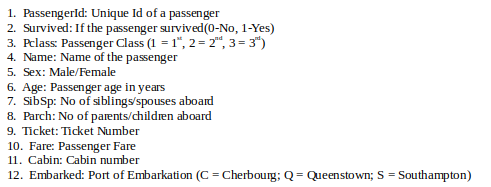`#importing pandas library ` `import` `pandas as pd ` ` `  `#loading data ` `titanic ``=` `pd.read_csv(``'...\input\train.csv'``) `

Seaborn:
It is a python library used to statistically visualize data. Seaborn, built over Matplotlib, provides a better interface and ease of usage. It can be installed using the following command,
`pip3 install seaborn`

 `# View first five rows of the dataset ` `titanic.head() `

Output :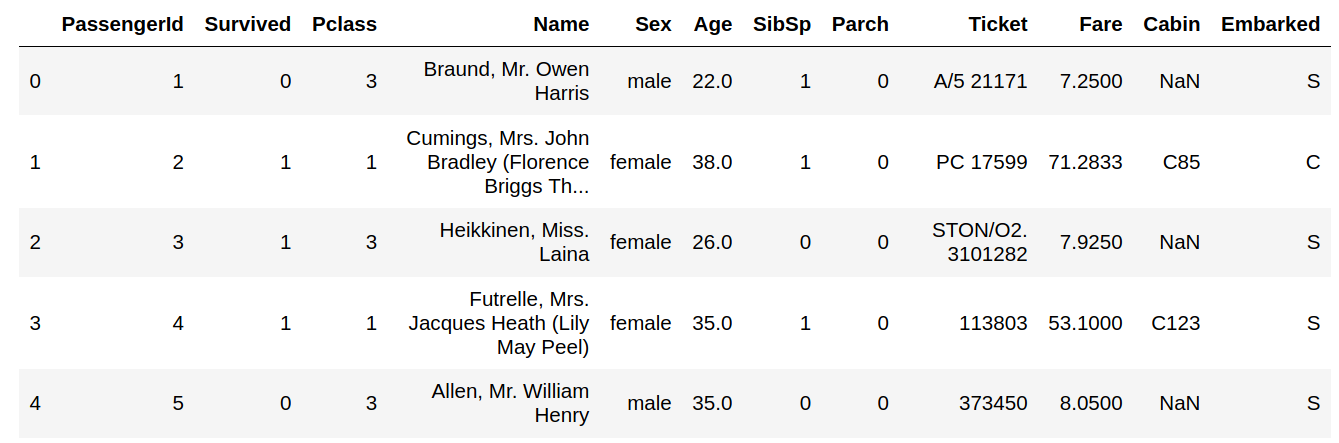Code : Checking the NULL values

 `titanic.isnull().``sum``() `

Output :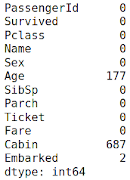The columns having null values are: Age, Cabin, Embarked. They need to be filled up with appropriate values later on.

Features: The titanic dataset has roughly the following types of features:

• Categorical/Nominal: Variables that can be divided into multiple categories but having no order or priority.
Eg. Embarked (C = Cherbourg; Q = Queenstown; S = Southampton)
• Binary: A subtype of categorical features, where the variable has only two categories.
Eg: Sex (Male/Female)
• Ordinal: They are similar to categorical features but they have an order(i.e can be sorted).
Eg. Pclass (1, 2, 3)
• Continuous: They can take up any value between the minimum and maximum values in a column.
Eg. Age, Fare
• Count: They represent the count of a variable.
Eg. SibSp, Parch
• Useless: They don’t contribute to the final outcome of an ML model. Here, PassengerId, Name, Cabin and Ticket might fall into this category.

Code : Graphical Analysis

 `import` `seaborn as sns ` `import` `matplotlib.pyplot as plt ` ` `  `# Countplot ` `sns.catplot(x ``=``"Sex"``, hue ``=``"Survived"``,  ` `kind ``=``"count"``, data ``=` `titanic) `

Output :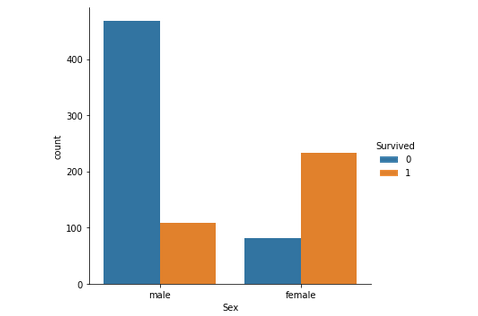Just by observing the graph, it can be approximated that the survival rate of men is around 20% and that of women is around 75%. Therefore, whether a passenger is a male or a female plays an important role in determining if one is going to survive.

Code : Pclass (Ordinal Feature) vs Survived

 `# Group the dataset by Pclass and Survived and then unstack them ` `group ``=` `titanic.groupby([``'Pclass'``, ``'Survived'``]) ` `pclass_survived ``=` `group.size().unstack() ` ` `  `# Heatmap - Color encoded 2D representation of data. ` `sns.heatmap(pclass_survived, annot ``=` `True``, fmt ``=``"d"``) `

Output: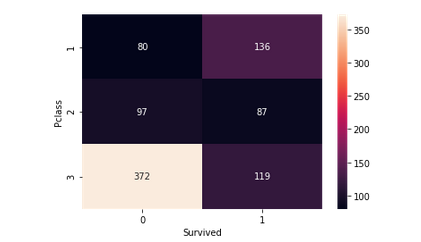It helps in determining if higher-class passengers had more survival rate than the lower class ones or vice versa. Class 1 passengers have a higher survival chance compared to classes 2 and 3. It implies that Pclass contributes a lot to a passenger’s survival rate.

Code : Age (Continuous Feature) vs Survived

 `# Violinplot Displays distribution of data  ` `# across all levels of a category. ` `sns.violinplot(x ``=``"Sex"``, y ``=``"Age"``, hue ``=``"Survived"``,  ` `data ``=` `titanic, split ``=` `True``) `

Output :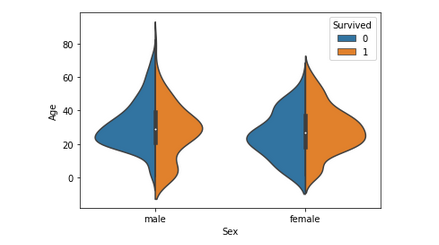This graph gives a summary of the age range of men, women and children who were saved. The survival rate is –

• Good for children.
• High for women in the age range 20-50.
• Less for men as the age increases.

Since Age column is important, the missing values need to be filled, either by using the Name column(ascertaining age based on salutation – Mr, Mrs etc.) or by using a regressor.
After this step, another column – Age_Range (based on age column) can be created and the data can be analyzed again.

Code : Factor plot for Family_Size (Count Feature) and Family Size.

 `# Adding a column Family_Size ` `titanic[``'Family_Size'``] ``=` `0` `titanic[``'Family_Size'``] ``=` `titanic[``'Parch'``]``+``titanic[``'SibSp'``] ` ` `  `# Adding a column Alone ` `titanic[``'Alone'``] ``=` `0` `titanic.loc[titanic.Family_Size ``=``=` `0``, ``'Alone'``] ``=` `1` ` `  `# Factorplot for Family_Size ` `sns.factorplot(x ``=``'Family_Size'``, y ``=``'Survived'``, data ``=` `titanic) ` ` `  `# Factorplot for Alone ` `sns.factorplot(x ``=``'Alone'``, y ``=``'Survived'``, data ``=` `titanic) `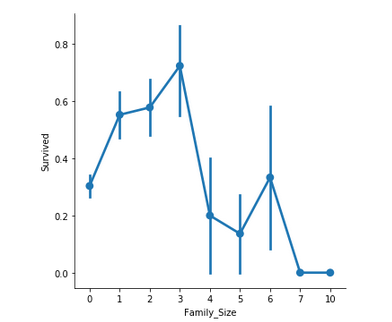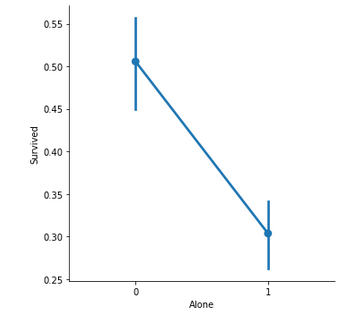Family_Size denotes the number of people in a passenger’s family. It is calculated by summing the SibSp and Parch columns of a respective passenger. Also, another column Alone is added to check the chances of survival of a lone passenger against the one with a family.

Important observations –

• If a passenger is alone, the survival rate is less.
• If the family size is greater than 5, chances of survival decreases considerably.

Code : Bar Plot for Fare (Continuous Feature)

 `# Divide Fare into 4 bins ` `titanic[``'Fare_Range'``] ``=` `pd.qcut(titanic[``'Fare'``], ``4``) ` ` `  `# Barplot - Shows approximate values based  ` `# on the height of bars. ` `sns.barplot(x ``=``'Fare_Range'``, y ``=``'Survived'``,  ` `data ``=` `titanic) `

Output :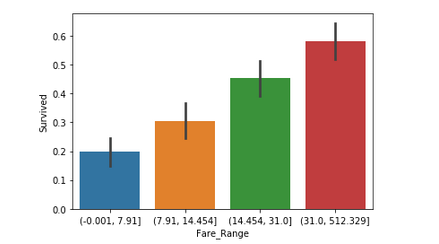Fare denotes the fare paid by a passenger. As the values in this column are continuous, they need to be put in separate bins(as done for Age feature) to get a clear idea. It can be concluded that if a passenger paid a higher fare, the survival rate is more.

Code : Categorical Count Plots for Embarked Feature

 `# Countplot ` `sns.catplot(x ``=``'Embarked'``, hue ``=``'Survived'``,  ` `kind ``=``'count'``, col ``=``'Pclass'``, data ``=` `titanic) `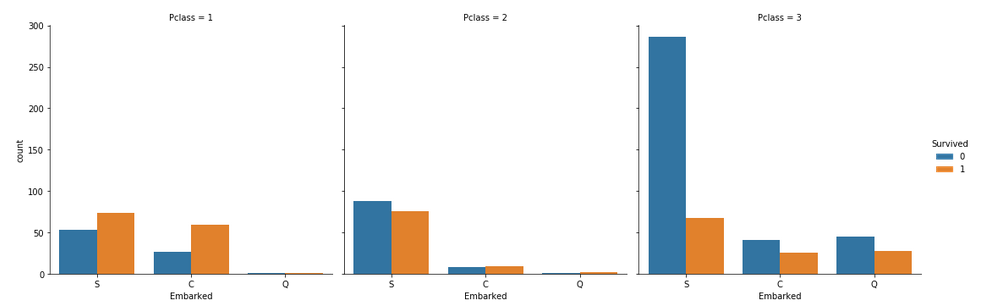Some notable observations are:

• Majority of the passengers boarded from S. So, the missing values can be filled with S.
• Majority of class 3 passengers boarded from Q.
• S looks lucky for class 1 and 2 passengers compared to class 3.

Conclusion :

• The columns that can be dropped are:
• PassengerId, Name, Ticket, Cabin: They are strings, cannot be categorized and don’t contribute much to the outcome.
• Age, Fare: Instead, the respective range columns are retained.
• The titanic data can be analyzed using many more graph techniques and also more column correlations, than, as described in this article.
• Once the EDA is completed, the resultant dataset can be used for predictions.

Attention geek! Strengthen your foundations with the Python Programming Foundation Course and learn the basics.

To begin with, your interview preparations Enhance your Data Structures concepts with the Python DS Course.

My Personal Notes arrow_drop_upCheck out this Author's contributed articles.

If you like GeeksforGeeks and would like to contribute, you can also write an article using contribute.geeksforgeeks.org or mail your article to contribute@geeksforgeeks.org. See your article appearing on the GeeksforGeeks main page and help other Geeks.

Please Improve this article if you find anything incorrect by clicking on the "Improve Article" button below.